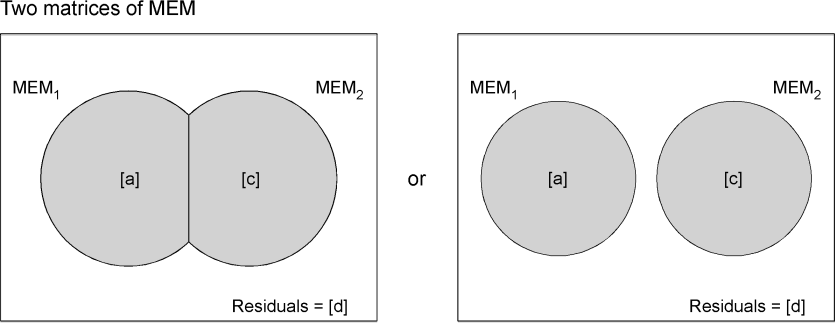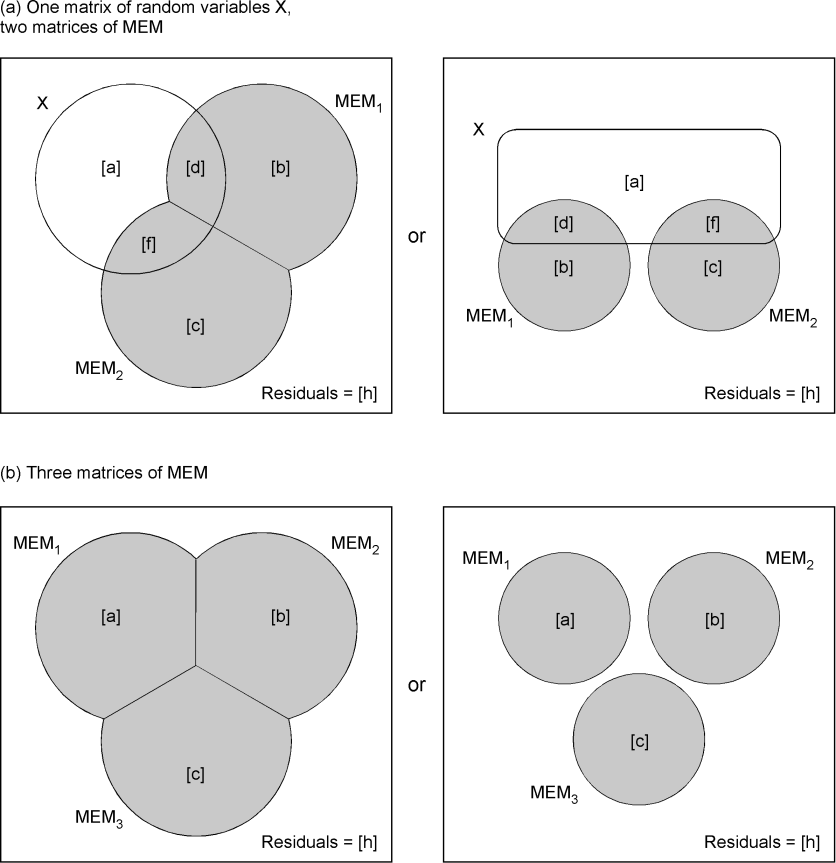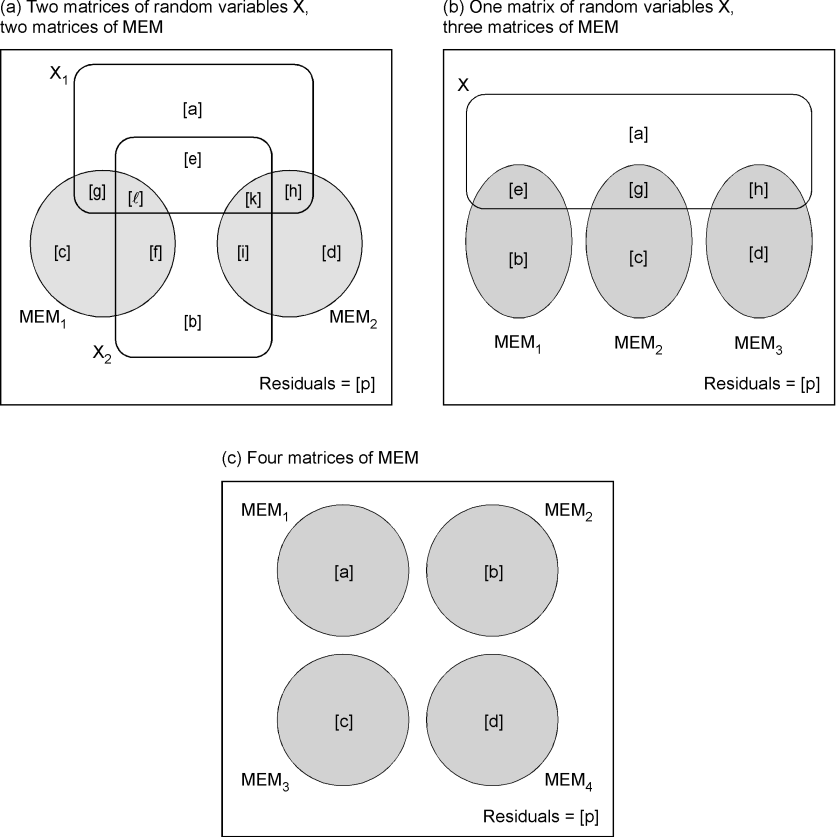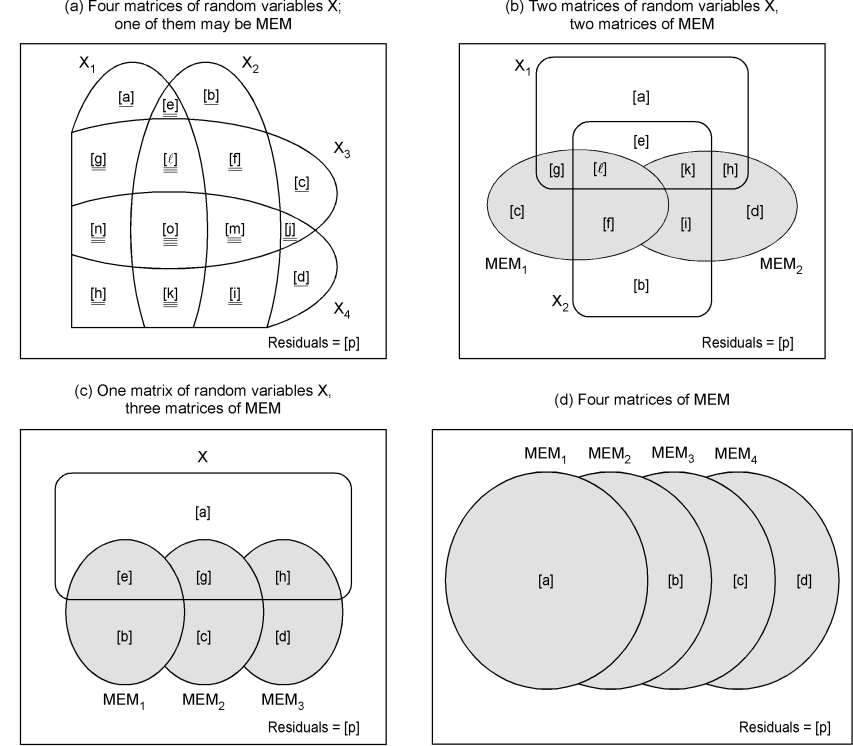Ecological Archives E093-106-A1

Pierre Legendre, Daniel Borcard, and David W. Roberts. 2012. Variation partitioning involving orthogonal spatial eigenfunction submodels. Ecology 93:1234–1240.

Appendix A. Variation partitioning formulas, hierarchical and proportional methods.

This appendix presents formulas for the calculation of fractions following the hierarchical and proportional variation partitioning methods for eigenfunction submodels (MEM or AEM). Classical variation partitioning (Borcard et al. 1992, Peres-Neto et al. 2006) only involves matrices of random variables. Different situations, involving different combinations of matrices of random (e.g. environmental) variables and spatial eigenfunction submodels, are described in Tables A1 to A3.

Venn diagrams illustrating the results of proportional apportioning of the shared fractions in variation partitioning are presented in Figs. A1 to A3. Figures illustrating the results of hierarchical partitioning of the shared fractions for two and three explanatory matrices are shown in the main paper, Figs. 1 and 2, and in Fig. A4 for four explanatory matrices.

Table A1. Variation partitioning for two explanatory matrices containing eigenfunction submodels. Fractions identifiers: see Figs. 1 and 2a. Notation: Ø is the empty set; in the present table, is denotes the absence of a value. Indications about the logic involved in the computation of some fractions follow the sign #.

 Hierarchical partitioning of the shared fraction [a] = [a] + [b] [b] = Ø # Transferred to [a] [c] = [c] [d] = [d] # Residual fraction Proportional apportioning of the shared fraction [a] = [a] + ([b] × [a] / ([a] + [c])) [b] = Ø # Transferred to [a] and [c] [c] = [c] + ([b] × [c] / ([a] + [c])) [d] = [d] # Residual fractionFig. A1. Venn diagrams illustrating the result of proportional apportioning of the shared fractions in variation partitioning involving two matrices of spatial eigenfunctions (MEM). Alternative graphical solutions are proposed. In gray, the fractions of variation explained by MEM.

Table A2. Variation partitioning in the 3-matrix case. Matrix 1 may contain random explanatory variables. (a) The last two, or (b) all three matrices, contain eigenfunction submodels. Fractions identifiers: see Fig. 3a. Notation as in Table A1.

 (a) Matrix 1 contains random explanatory variables matrices 2 and 3 contain eigenfunction submodels Hierarchical partitioning of the shared fractions (Fig. 3b) [a] = [a] [b] = [b] + [e] [c] = [c] [d] = [d] + [g] [e] = Ø # Transferred to [b] [f] = [f] [g] = Ø # Transferred to [d] [h] = [h] # Residual fraction Proportional apportioning of the shared fractions e.to.b = [e] × (([b] + [d]) / ([b] + [d] + [c] + [f]) # Portion of [e] transferred to [b] e.to.c = [e] × (([c] + [f]) / ([b] + [d] + [c] + [f]) # Portion of [e] transferred to [c] g.to.d = [g] × (([b] + [d]) / ([b] + [d] + [c] + [f]) # Portion of [g] transferred to [d] g.to.f = [g] × (([c] + [f]) / ([b] + [d] + [c] + [f]) # Portion of [g] transferred to [f] [a] = [a] [b] = [b] + e.to.b [c] = [c] + e.to.c [d] = [d] + g.to.d [e] = Ø # Transferred to [b] and [c] [f] = [f] + g.to.f [g] = Ø # Transferred to [d] and [f] [h] = [h] # Residual fraction (b) Three matrices of eigenfunction submodels Hierarchical partitioning of the shared fractions (Fig. 3c) [a] = [a] + [d] + [f] + [g] [b] = [b] + [e] [c] = [c] [d] = Ø # Transferred to [a] [e] = Ø # Transferred to [b] [f] = Ø # Transferred to [a] [g] = Ø # Transferred to [a] [h] = [h] # Residual fraction Proportional apportioning of the shared fractions d.to.a= [d] × ([a] / ([a] + [b]) # Portion of [d] transferred to [a] d.to.b= [d] × ([b] / ([a] + [b]) # Portion of [d] transferred to [b] e.to.b= [e] × ([b] / ([b] + [c]) # Portion of [e] transferred to [b] e.to.c = [e] × ([c] / ([b] + [c]) # Portion of [e] transferred to [c] f.to.a = [f] × ([a] / ([a] + [c]) # Portion of [f] transferred to [a] f.to.c = [f] × ([c] / ([a] + [c]) # Portion of [f] transferred to [c] g.to.a = [g] × ([a] / ([a] + [b] + [c]) # Portion of [g] transferred to [a] g.to.b = [g] × ([b] / ([a] + [b] + [c]) # Portion of [g] transferred to [b] g.to.c = [g] × ([c] / ([a] + [b] + [c]) # Portion of [g] transferred to [c] [a] = [a] + d.to.a + f.to.a + g.to.a [b] = [b] + d.to.b + e.to.b + g.to.b [c] = [c] + e.to.c + f.to.c + g.to.c [d] = Ø # Transferred to [a] and [b] [e] = Ø # Transferred to [b] and [c] [f] = Ø # Transferred to [a] and [c] [g] = Ø # Transferred to [a], [b] and [c] [h] = [h] # Residual fractionFig. A2. Venn diagrams illustrating the result of proportional apportioning of the shared fractions in variation partitioning involving (a) one matrix of random (e.g. environmental) variables and two matrices of spatial eigenfunctions (MEM), or (b) three matrices of spatial eigenfunctions (MEM). Alternative graphical solutions are proposed in each case. The fractions of variation explained by MEM matrices are in gray.

Table A3. Variation partitioning in the 4-matrix case, for matrices of random explanatory variables or matrices of eigenfunctions. Fractions identifiers: see Fig. A4a. Notation as in Table A1.

 (a) Matrices 1 and 2 contain random explanatory variables, matrices 3 and 4 contain eigenfunction submodels Hierarchical partitioning of the shared fractions (Fig. A4b) [a] = [a] [b] = [b] [c] = [c] + [j] [d] = [d] [e] = [e] [f] = [f] + [m] [g] = [g] + [n] [h] = [h] [i] = [i] [k] = [k] [l] = [l] + [o] [j] = Ø # Transferred to [c] [m] = Ø # Transferred to [f] [n] = Ø # Transferred to [g] [o] = Ø # Transferred to [l] [p] = [p] # Residual fraction Proportional apportioning of the shared fractions (Fig. A3a) j.to.c = [j] × [c + f + g + l] / ([c + f + g + l] + [d + h + i + k]) # Portion of [j] transferred to [c] j.to.d = [j] × [d + h + i + k] / ([c + f + g + l] + [d + h + i + k]) # Portion of [j] transferred to [d] m.to.f = [m] × [c + f + g + l] / ([c + f + g + l] + [d + h + i + k]) # Portion of [m] transferred to [f] m.to.i = [m] ×  [d + h + i + k] / ([c + f + g + l] + [d + h + i + k]) # Portion of [m] transferred to [i] n.to.g = [n] ×  [c + f + g + l] / ([c + f + g + l] + [d + h + i + k]) # Portion of [n] transferred to [g] n.to.h = [n] ×  [d + h + i + k] / ([c + f + g + l] + [d + h + i + k]) # Portion of [n] transferred to [h] o.to.k = [o] ×  [d + h + i + k] / ([c + f + g + l] + [d + h + i + k]) # Portion of [o] transferred to [k] o.to.l = [o] × [c + f + g + l] / ([c + f + g + l] + [d + h + i + k]) # Portion of [o] transferred to [l] [c] = [c] + j.to.c [d] = [d] + j.to.d [f] = [f] + m.to.f [g] = [g] + n.to.g [h] = [h] + n.to.h [i] = [i] + m.to.i [k] = [k] + o.to.k [l] = [l] + o.to.l [j] = Ø # Transferred to [c] and [d] [m] = Ø # Transferred to [f] and [i] [n] = Ø # Transferred to [g] and [h] [o] = Ø # Transferred to [k] and [l] [p] = [p] # Residual fraction (b) Matrix 1 contains random explanatory variables, matrices 2, 3 and 4 contain eigenfunction submodels Hierarchical partitioning of the shared fractions (Fig. A4c) [a] = [a] [b] = [b] + [f] + [i] + [m] [c] = [c] + [j] [d] = [d] [e] = [e] + [k] + [l] + [o] [g] = [g] + [n] [h] = [h] [f] = [i] = [j] = [k] = [l] = [m] = [n] = [o] = Ø # Transferred to other fractions [p] = [p] Proportional apportioning of the shared fractions (Fig. A3b) f.to.b = [f] × [b + e] / ([b + e] + [c + g]) # Portion of [f] transferred to [b] f.to.c = [f] × [c + g] / ([b + e] + [c + g]) # Portion of [f] transferred to [c] i.to.b = [i] × [b + e] / ([b + e] + [d + h]) # Portion of [i] transferred to [b] i.to.d = [i] × [d + h] / ([b + e] + [d + h]) # Portion of [ji] transferred to [d] j.to.c = [j] × [c + g] / ([c + g] + [d + h]) # Portion of [j] transferred to [c] j.to.d = [j] × [d + h] / ([c + g] + [d + h]) # Portion of [j] transferred to [d] k.to.e = [k] × [b + e] / ([b + e] + [d + h]) # Portion of [k] transferred to [e] k.to.h = [k] × [d + h] / ([b + e] + [d + h]) # Portion of [k] transferred to [h] l.to.e = [l] × [b + e] / ([b + e] + [c + g]) # Portion of [l] transferred to [e] l.to.g = [l] × [c + g] / ([b + e] + [c + g]) # Portion of [l] transferred to [g] m.to.b = [m] × [b + e] / ([b + e] + [c + g] + [d + h]) # Portion of [m] transferred to [b] m.to.c = [m] × [c + g] / ([b + e] + [c + g] + [d + h]) # Portion of [m] transferred to [c] m.to.d = [m] × [d + h] / ([b + e] + [c + g] + [d + h]) # Portion of [m] transferred to [d] n.to.g = [n] × [c + g] / ([c + g] + [d + h]) # Portion of [n] transferred to [g] n.to.h = [n] × [d + h] / ([c + g] + [d + h]) # Portion of [n] transferred to [h] o.to.e = [o] × [b + e] / ([b + e] + [c + g] + [d + h]) # Portion of [o] transferred to [e] o.to.g = [o] × [c + g] / ([b + e] + [c + g] + [d + h]) # Portion of [o] transferred to [g] o.to.h = [o] × [d + h] / ([b + e] + [c + g] + [d + h]) # Portion of [o] transferred to [h] [b] = [b] + f.to.b + m.to.b + i.to.b [c] = [c] + j.to.c + f.to.c + m.to.c [d] = [d] + j.to.d + i.to.d + m.to.d [e] = [e] + k.to.e + l.to.e + o.to.e [g] = [g] + l.to.g + n.to.g + o.to.g [h] = [h] + k.to.h + n.to.h + o.to.h [f] = [i] = [j] = [k] = [l] = [m] = [n] = [o] = Ø # Transferred to other fractions [p] = [p] # Residual fraction (c) Four matrices of eigenfunction submodels Hierarchical partitioning of the shared fractions (Fig. A4d) [a] = [a] + [e] + [g] + [h] + [k] + [l] + [n] + [o] [b] = [b] + [f] + [i] + [m] [c] = [c] + [j] [d] = [d] [e] = [f] = [g] = [h] = [i] = [j] = [k] = [l] = [m] = [n] = [o] = Ø # Transferred to other fractions [p] = [p] ] # Residual fraction Proportional apportioning of the shared fractions (Fig. A3c) e.to.a = [e] × [a] / ([a] + [b]) # Portion of [e] transferred to [a] e.to.b = [e] × [b] / ([a] + [b]) # Portion of [e] transferred to [b] f.to.b = [f] × [b] / ([b] + [c]) # Portion of [f] transferred to [b] f.to.c = [f] × [c] / ([b] + [c]) # Portion of [f] transferred to [c] g.to.a = [g] × [a] / ([a] + [c]) # Portion of [g] transferred to [a] g.to.c = [g] × [c] / ([a] + [c]) # Portion of [g] transferred to [c] h.to.a = [h] × [a] / ([a] + [d]) # Portion of [h] transferred to [a] h.to.d = [h] × [d] / ([a] + [d]) # Portion of [h] transferred to [d] i.to.b = [i] × [b] / ([b] + [d]) # Portion of [i] transferred to [b] i.to.d = [i] × [d] / ([b] + [d]) # Portion of [i] transferred to [d] j.to.c = [j] × [c] / ([c] + [d]) # Portion of [j] transferred to [c] j.to.d = [j] × [d] / ([c] + [d]) # Portion of [j] transferred to [d] k.to.a = [k] × [a] / ([a] + [b] + [d]) # Portion of [k] transferred to [a] k.to.b = [k] × [b] / ([a] + [b] + [d]) # Portion of [k] transferred to [b] k.to.d = [k] × [d] / ([a] + [b] + [d]) # Portion of [k] transferred to [d] l.to.a = [l] × [a] / ([a] + [b] + [c]) # Portion of [l] transferred to [a] l.to.b = [l] × [b] / ([a] + [b] + [c]) # Portion of [l] transferred to [b] l.to.c = [l] × [c] / ([a] + [b] + [c]) # Portion of [l] transferred to [c] m.to.b = [m] × [b] / ([b] + [c] + [d]) # Portion of [m] transferred to [b] m.to.c = [m] × [c] / ([b] + [c] + [d]) # Portion of [m] transferred to [c] m.to.d = [m] × [d] / ([b] + [c] + [d]) # Portion of [m] transferred to [d] n.to.a = [n] × [a] / ([a] + [c] + [d]) # Portion of [n] transferred to [a] n.to.c = [n] × [c] / ([a] + [c] + [d]) # Portion of [n] transferred to [c] n.to.d = [n] × [d] / ([a] + [c] + [d]) # Portion of [n] transferred to [d] o.to.a = [o] × [a] / ([a] + [b] + [c] + [d]) # Portion of [o] transferred to [a] o.to.b = [o] × [b] / ([a] + [b] + [c] + [d]) # Portion of [o] transferred to [b] o.to.c = [o] × [c] / ([a] + [b] + [c] + [d]) # Portion of [o] transferred to [c] o.to.d = [o] × [d] / ([a] + [b] + [c] + [d]) # Portion of [o] transferred to [d] [a] = [a] + e.to.a + g.to.a + h.to.a + k.to.a + l.to.a + n.to.a + o.to.a [b] = [b] + e.to.b + f.to.b + i.to.b + k.to.b + l.to.b + m.to.b + o.to.b [c] = [c] + f.to.c + g.to.c + j.to.c + l.to.c + m.to.c + n.to.c + o.to.c [d] = [d] + h.to.d + i.to.d + j.to.d + k.to.d + m.to.d + n.to.d + o.to.d [e] = [f] = [g] = [h] = [i] = [j] = [k] = [l] = [m] = [n] = [o] = Ø # Transferred to other fractions [p] = [p] ] # Residual fractionFig. A3. Venn diagrams illustrating the result of proportional apportioning of the shared fractions in variation partitioning involving (a) two matrices of random (e.g. environmental) variables and two matrices of spatial eigenfunctions (MEM), (b) one matrix of random (e.g. environmental) variables and three matrices of spatial eigenfunctions (MEM), or (c) four matrices of spatial eigenfunctions (MEM). The fractions of variation explained by MEM matrices are in gray.Fig. A4. Venn diagrams of variation partitioning results for four explanatory matrices. (a) Classical partitioning; the number of lines underneath the fraction identifiers corresponds to the number of explanatory matrices X they intersect. (b, c, d) Result of hierarchical partitioning.

Literature Cited

Borcard, D., P. Legendre, and P. Drapeau. 1992. Partialling out the spatial component of ecological variation. Ecology 73:1045–1055.

Peres-Neto, P. R., P. Legendre, S. Dray, and D. Borcard. 2006. Variation partitioning of species data matrices: estimation and comparison of fractions. Ecology 87:2614–2625.